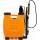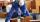Strawberries

Father collects strawberries himself in 4 hours, son in 14 hours. How long will it take them along when dad comes to help son collect strawberries after 3 hours?

Result

x =  2.444 h

Solution:

x/4+(x+3)/14=1

9x = 22

x = 229 ≈ 2.444444

Calculated by our simple equation calculator.

Leave us a comment of this math problem and its solution (i.e. if it is still somewhat unclear...):Be the first to comment!To solve this verbal math problem are needed these knowledge from mathematics:

Need help calculate sum, simplify or multiply fractions? Try our fraction calculator. Do you have a linear equation or system of equations and looking for its solution? Or do you have quadratic equation?

Next similar examples:

1. TogetherGrandfather, father, and son are carpenters. They decided to make a wardrobe. Father produces it for 12 hours, his son for 15 hours, and grandfather for 10 hours. How long will they make it together?
2. SprayersThe vineyard can be sprayed first sprayer in 5 hours and with the second in 8 hours. How long would it take if we use sprayers both at once?
3. Pumps A and BPump A fill the tank for 12 minutes, pump B for 24 minutes. How long will take to fill the tank if he is only three minutes works A and then both pumps A and B?
4. Construction of roadFor the construction of the road dam, it is supposed to transport six trucks a total of 18 days. After three days, two more cars were sent to the construction site. How long will it take to carry the material?
5. Hectoliters of waterThe pool has a total of 126 hectoliters of water. The first pump draws 2.1 liters of water per second. A second pump pumps 3.5 liters of water per second. How long will it take both pumps to drain four-fifths of the water at the same time?
6. PlayingHow long have we trained on the pitch when we know that the warm-up took 10 minutes, we trained passes for one-third of the time and we played football half the time?
7. Six yearsIn six years Jan will be twice as old as he was six years ago. How old is he?
8. PearsThere were pears in the basket, I took two-fifths of them, and left six in the basket. How many pears did I take?
9. FractionsThree-quarters of an unknown number are 4/5. What is 5/6 of this unknown number?
10. Bricklayers8 bricklayers build a house for 630 days. How many bricklayers have to add after 150 days, so then the whole building completed in (next) 320 days?
11. Equation with fractionsSolve equation: ? It is equation with fractions.
12. Equation with xSolve the following equation: 2x- (8x + 1) - (x + 2) / 5 = 9
13. Fraction + eqSolve following simple equation with fractions: -5/6(8+5b) = 75 + 5/3b
14. EquationSolve the equation: 1/2-2/8 = 1/10; Write the result as a decimal number.
15. Unknown numberI think the number - its sixth is 3 smaller than its third.
16. Eq-fracSolve the following equation with fractions: h + 1/3 =5/3
17. EqnSolve equation with fractions: 2x/3-50=40+x/4